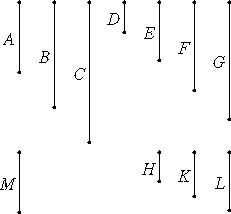# Proposition 33

Given as many numbers as we please, to find the least of those which have the same ratio with them.

Let A, B, and C be the given numbers, as many as we please.

It is required to find the least of those which have the same ratio with A, B, and C.

Either A, B, and C are relatively prime or they are not.VII.21

Now, if A, B, and C are relatively prime, then they are the least of those which have the same ratio with them.

VII.3

But, if not, take D the greatest common measure of A, B, and C. Let there be as many units in the numbers E, F, and G as the times that D measures the numbers A, B, and C respectively.

Therefore the numbers E, F, and G measure the numbers A, B, and C respectively according to the units in D. Therefore E, F, and G measure A, B, and C the same number of times. Therefore E, F, and G are in the same ratio with A, B, and C.

I say next that they are the least that are in that ratio.

If E, F, and G are not the least of those which have the same ratio with A, B, and C, then there are numbers less than E, F, and G in the same ratio with A, B, and C. Let them be H, K, and L. Therefore H measures A the same number of times that the numbers K and L measure the numbers B and C respectively.

Let there be as many units in M as the times that H measures A. Then the numbers K and L also measure the numbers B and C respectively according to the units in M.

VII.16

And, since H measures A according to the units in M, therefore M also measures A according to the units in H. For the same reason M also measures the numbers B and C according to the units in the numbers K and L respectively. Therefore M measures A, B, and C.

VII.Def.15

Now, since H measures A according to the units in M, therefore H multiplied by M makes A. For the same reason also E multiplied by D makes A.

VII.19

Therefore the product of E and D equals the product of H and M. Therefore E is to H as M is to D.

But E is greater than H, therefore M is also greater than D. And it measures A, B, and C, which is impossible, for by hypothesis, D is the greatest common measure of A, B, and C.

Therefore there cannot be any numbers less than E, F, and G which are in the same ratio with A, B, and C. Therefore E, F, and G are the least of those which have the same ratio with A, B, and C.

Q.E.D.

## Guide

#### The beginning of the proof

Given three numbers a, b, etc., let d be their greatest common divisor. If d measures a m times, let e = mu, where u is the unit. Likewise, if b = nd, let f = nb, etc. (Euclid hasn’t defined division, but he’s essentially saying let e = a/d, f = b/d, etc.) Then a = kd, b = ke, etc., where d = ku. Therefore, a : b : c = e : f : g.

The remainder of the proof shows that no smaller numbers are in the same ratio.

#### Multiple term ratios

This proposition is unusual in that it discusses a ratio a : b : c of three (or more) numbers. It also has the proportion a : b : c = e : f : g. These multiterm ratios and proportions may have been left over from an earlier time. Euclid argues that the proportion holds because e, f, and g measure a, b, and c, respectively, the same number, d, times. By the definition of proportion, that observation directly implies

a : e = b : f = c : g

The desired proportion, a : b : c = e : f : g is an alternate form of that multiple proportion. See V.Def.13 for the definition of alternate ratios.

#### Use of this proposition

This proposition is used in the next one and several propositions in Book VIII starting with VIII.6.